# High School Math : Finding Definite Integrals

## Example Questions

1 2 4 Next →

### Example Question #31 : Finding Integrals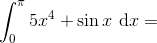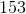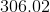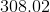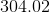Explanation:

To solve this problem we can use the Fundamental Theorem of Calculus: If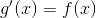, then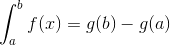.

First we need to find the indefinite integral.

Since we're adding terms, we take the integral or anti-derivative of each part separately. For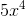, we can use the reverse power rule, which states that we raise the exponent of the variable by one and then divide by that new exponent. For sine, we use our trigonometric integral rules.

Remember,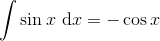.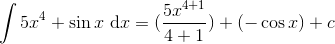Don't forget to include a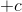to account for any constants!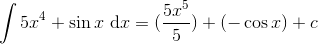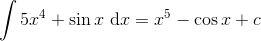Plug that into the FTOC: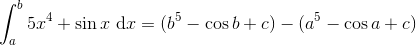Notice that the's cancel out.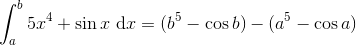Plug in our given values: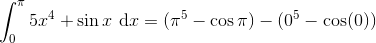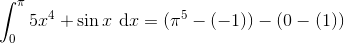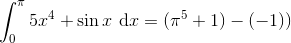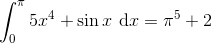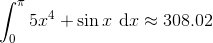### Example Question #51 : Calculus Ii — Integrals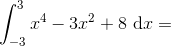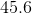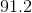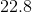Explanation:

To find the definite integral, we can use the Fundamental Theorem of Calculus. This states that if, then.

To use the FToC, we need to find our indefinite integral of our given equation.

To find the indefinite integral, or anti-derivative, we can use the reverse power rule. We raise the exponent of each variable by one and divide by that new exponent.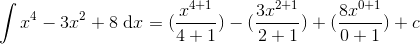Don't forget to include ato cover any constant!

Simplify.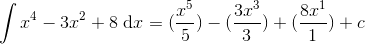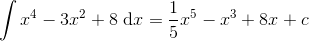We can now plug that into FToC!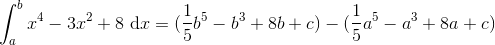Notice that the's cancel out.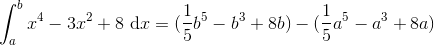Plug in our given values.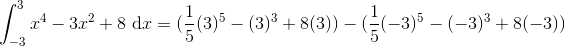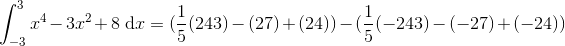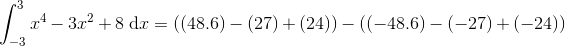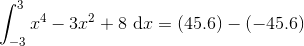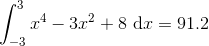### Example Question #31 : Finding Definite Integrals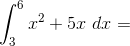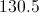Undefined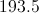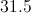Explanation:

Remember the Rundamental Theorem of Calculus: If, then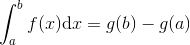.

To solve for the indefinite integral, we can use the reverse power rule. We raise the power of the exponents by one and divide by that new exponent. For this problem, that would look like: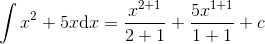Remember, when taking an integral, definite or indefinite, we always add, as there could be a constant involved.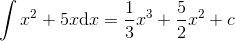Now we can plug that back into the problem.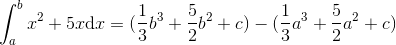Notice that the's cancel out. Plug in the values given in the problem: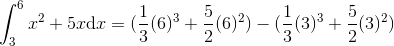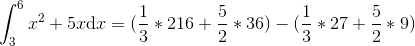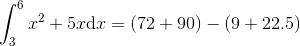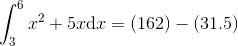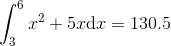1 2 4 Next →

### All High School Math Resources